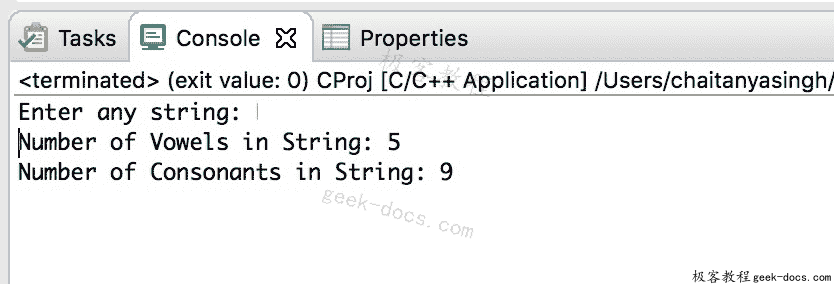# C 程序 计算元音和辅音

#include <stdio.h>
int main()
{
char str;
char *p;
int  vCount=0,cCount=0;

printf("Enter any string: ");
fgets(str, 100, stdin);

//assign base address of char array to pointer
p=str;

//'\0' signifies end of the string
while(*p!='\0')
{
if(*p=='A' ||*p=='E' ||*p=='I' ||*p=='O' ||*p=='U'
||*p=='a' ||*p=='e' ||*p=='i' ||*p=='o' ||*p=='u')
vCount++;
else
cCount++;
//increase the pointer, to point next character
p++;
}

printf("Number of Vowels in String: %d\n",vCount);
printf("Number of Consonants in String: %d",cCount);
return 0;
}• 回顶